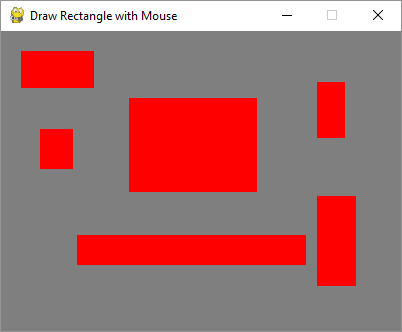# Pygame - Moving Rectangular Objects

The Pygame.Rect class has functionality to store and manipulate rectangular areas. A Rect object can be constructed from left, top, width and height values. Functions in Rect class enable copying, moving nd resizing the Rect object.

A Rect object has following virtual attributes −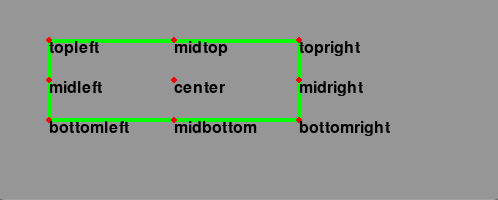In addition to movement, Rect class has methods to test collision between rectangles.

 copy() Returns a new rectangle having the same position and size as the original. move() Returns a new rectangle that is moved by the given offset. The x and y arguments can be any integer value, positive or negative. move_ip() Same as the Rect.move() method, but operates in place. inflate(x,y) Returns a new rectangle with the size changed by the given offset. Negative values will shrink the rectangle. inflate_ip(x, y) Same as the Rect.inflate() method, but operates in place. clamp(Rect) Returns a new rectangle that is moved to be completely inside the argument Rect. clip(Rect) Returns a new rectangle that is cropped to be completely inside the argument Rect. union(Rect) Returns a new rectangle that completely covers the area of the two provided rectangles. union_ip(Rect) Same as the Rect.union() method, but operates in place. contains(Rect) Returns true when the argument is completely inside the Rect. collidepoint((x,y)) Returns true if the given point is inside the rectangle. colliderect(Rect) Returns true if any portion of either rectangle overlap

## Example

In the following program, a Rect object is drawn with red outline. Using copy() method, its clone is created for movement. The movement is effected by move_ip() method. The arrow keys move the position of copied rectangle by incrementing/decrementing x/y coordinate by + or -5 pixels.

import pygame
from pygame.locals import *
from sys import exit
pygame.init()
screen = pygame.display.set_mode((400,300))
rect1 = Rect(50, 60, 200, 80)
rect2=rect1.copy()
running = True
x=0
y=0
while running:
for event in pygame.event.get():
if event.type == QUIT:
running = False
if event.type == KEYDOWN:
if event.key==K_LEFT:
x= -5
y=0
if event.key == K_RIGHT:
x=5
y=0
if event.key == K_UP:
x = 0
y = -5
if event.key == K_DOWN:
x = 0
y = 5
rect2.move_ip(x,y)
screen.fill((127,127,127))
pygame.draw.rect(screen, (255,0,0), rect1, 1)
pygame.draw.rect(screen, (0,0,255), rect2, 5)
pygame.display.update()
pygame.quit()


## Output

The following output shows rectangle with red outline is the original rectangle. Its copy keeps moving responding to arrow keys and has blue outline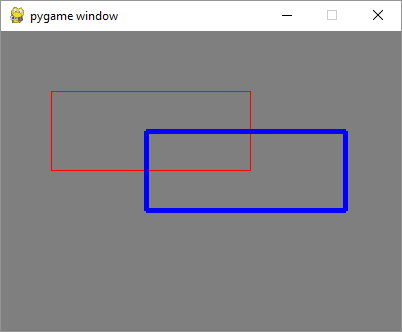## Example

Changing the move_ip() method to inflate_ip() method to grow/shrink the rectangle depending upon the arrow pressed.

while running:
for event in pygame.event.get():
if event.type == QUIT:
running = False
if event.type == KEYDOWN:
if event.key==K_LEFT:
x= -5
y=0
if event.key == K_RIGHT:
x=5
y=0
if event.key == K_UP:
x = 0
y = -5
if event.key == K_DOWN:
x = 0
y = 5
rect2.inflate_ip(x,y)
screen.fill((127,127,127))
pygame.draw.rect(screen, (255,0,0), rect1, 1)
pygame.draw.rect(screen, (0,0,255), rect2, 5)
pygame.display.update()


## Output

The following is the screenshot of the arrow key-press activity −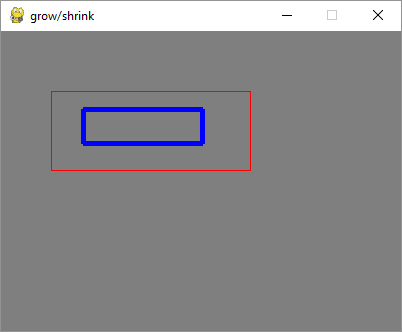## Example

To make the rectangle move by detecting MOUSEMOTION event, we need to first press the mouse inside the original rectangle. To verify whether mouse position is inside the rectangle, we use collidepoint() method of the Rect object. While the mouse is in motion, the rectangle object is moved in place by move_ip() method. Movement shall stop when mouse is released.

import pygame
from pygame.locals import *
from sys import exit
pygame.init()
screen = pygame.display.set_mode((400,300))
rect = Rect(50, 60, 200, 80)
moving = False
running = True
while running:
for event in pygame.event.get():
if event.type == QUIT:
running = False
elif event.type == MOUSEBUTTONDOWN:
if rect.collidepoint(event.pos):
moving = True
elif event.type == MOUSEBUTTONUP:
moving = False
elif event.type == MOUSEMOTION and moving:
rect.move_ip(event.rel)
screen.fill((127,127,127))
pygame.draw.rect(screen, (255,0,0), rect)
if moving:
pygame.draw.rect(screen, (0,0,255), rect, 4)
pygame.display.flip()
pygame.quit()


## Output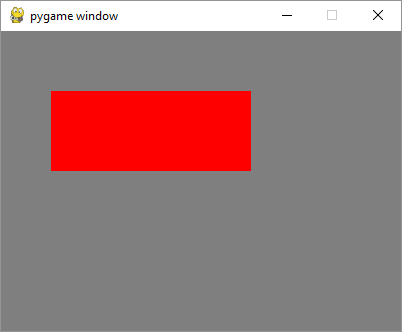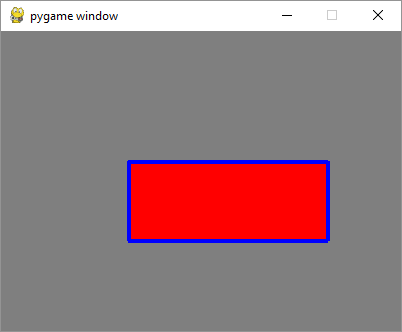## Example

To draw rectangle by mouse, capture the mouse pointer coordinates in MOUSEBUTTONDOWN and MOUSEBUTTONUP events, calculate the topleft coordinates, width and height and call rect() function.

import pygame
from pygame.locals import *
from sys import exit
pygame.init()
screen = pygame.display.set_mode((400,300))
pygame.display.set_caption("Draw Rectangle with Mouse")
screen.fill((127,127,127))
x=0
y=0
w=0
h=0
drawmode=True
running = True
while running:
for event in pygame.event.get():
if event.type == QUIT:
running = False
if event.type == MOUSEBUTTONDOWN:
x,y = pygame.mouse.get_pos()
drawmode = True
if event.type == MOUSEBUTTONUP:
x1,y1 = pygame.mouse.get_pos()
w=x1-x
h=y1-y
drawmode= False

rect = pygame.Rect(x,y,w,h)
if drawmode == False:
pygame.draw.rect(screen, (255,0,0), rect)
pygame.display.flip()
pygame.quit()


## Output